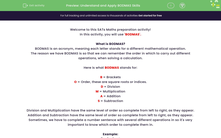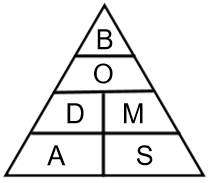# Understand and Apply BODMAS Skills

In this worksheet, students will apply BODMAS skills in order to solve a range of calculations. BODMAS is an acronym designed to help students understand the order in which to complete a calculation.This content is premium and exclusive to EdPlace subscribers.Key stage:  KS 2

Curriculum topic:   Exam-style Questions: SATs Maths

Curriculum subtopic:   Exam-Style Questions: BODMAS Skills

Difficulty level:#### Worksheet Overview

Welcome to this SATs Maths preparation activity!

In this activity, you will use 'BODMAS'.

What is BODMAS?

BODMAS is an acronym, meaning each letter stands for a different mathematical operation.

The reason we have BODMAS is so that we can remember the order in which to carry out different operations, when solving a calculation.

Here is what BODMAS stands for:

B = Brackets

O = Order, these are square roots or indices.

D = Division

M = Multiplication

S = Subtraction

Sometimes, you may see 'BODMAS' called 'BIDMAS' - it is exactly the same, but the 'I' stands for Indices.

As you can see in the pyramid below, Division and Multiplication have the same level of order so complete from left to right, as they appear.

Addition and Subtraction have the same level of order as well, so complete from left to right, as they appear.Sometimes, we have to complete a number sentence with several different operations in so it's very important to know which order to complete them in.

Example:

7 + 8 x 3 = ?

BODMAS

Step 1: Complete the multiplication first = 8 x 3 = 24

Step 2: Next complete the addition = 7 + 24 = 31